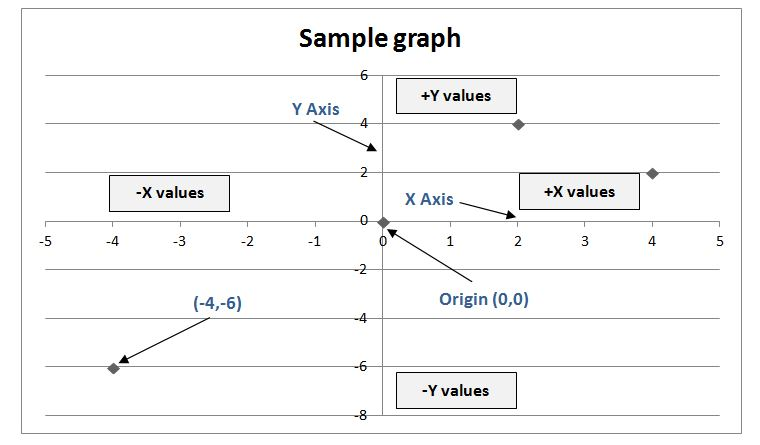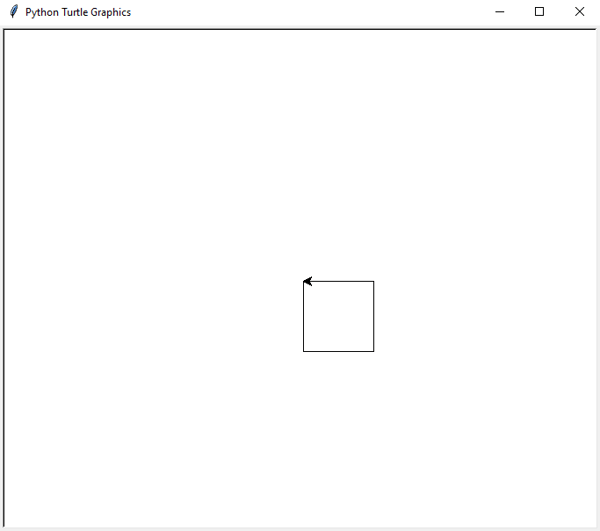Related Tags

graph
basics
fundamentals
communitycreator

# Graph fundamentals

Chetana Tukkoji

A graph is a set of vertices connected to each other. It has at least one line joining a set of two vertices with no vertex connecting itself. Some basic terms are:

• point
• line
• vertex
• edge
• degree of vertices
• properties of graphs
• etc.### Point

A point is a particular position in a one-dimensional, two-dimensional, or three-dimensional space. For better understanding, a point can be denoted by a letter and represented with a dot. ​

#### For example

The graph on the right shows the dot as a point named ‘a’.### Line

A line is a connection between two points. It can be represented with a solid line

#### For example:

The graph on the right shows points ‘a’ and ‘b’. The link between these two points is called a line.graph = { "a" : ["c"],
"b" : ["c", "e"],
"c" : ["a", "b", "d", "e"],
"d" : ["c"],
"e" : ["c", "b"],
"f" : []
}

def generate_edges(graph):
edges = []
for node in graph:
for neighbour in graph[node]:
edges.append((node, neighbour))

return edges

print(generate_edges(graph))

Graphical representation of the output

## Vertex

A vertex is a point where multiple lines meet. It is also called a node. Similar to points, a vertex is denoted by a​ letter.

## Edge

An edge is a mathematical term used for a line that connects two vertices. Many edges can be formed from a single vertex. However, without a vertex, an edge cannot be formed – t​here must be a starting vertex and an ending vertex for an edge.

## Graph

A graph, G , is defined as G = (V, E) – where V is a set of all vertices and E is a set of all edges in the graph.### Loop

In a graph, if an edge is drawn from the vertex to itself, it is called a loop. In the illustration, V is a vertex whose edge, (V, V), is forming a loop.## For example

Turtle is a built-in module in Python. It provides you with a drawing canvas (cardboard) and turtle (pen). To draw something on the canvas, we need to move the turtle (pen). To move the turtle, there are some functions:

• forward()
• backward()
• left()
• right()
import turtle

t = turtle.Turtle()

t.right(90)
t.forward(80)

t.left(90)
t.forward(80)

t.left(90)
t.forward(80)

t.left(90)
t.forward(80)RELATED TAGS

graph
basics
fundamentals
communitycreator

CONTRIBUTOR

Chetana Tukkoji
RELATED COURSES

View all Courses

Keep Exploring

Learn in-demand tech skills in half the time